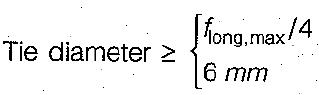Courses

# Test: Columns - 2

## 10 Questions MCQ Test Topicwise Question Bank for Civil Engineering | Test: Columns - 2

Description
This mock test of Test: Columns - 2 for Civil Engineering (CE) helps you for every Civil Engineering (CE) entrance exam. This contains 10 Multiple Choice Questions for Civil Engineering (CE) Test: Columns - 2 (mcq) to study with solutions a complete question bank. The solved questions answers in this Test: Columns - 2 quiz give you a good mix of easy questions and tough questions. Civil Engineering (CE) students definitely take this Test: Columns - 2 exercise for a better result in the exam. You can find other Test: Columns - 2 extra questions, long questions & short questions for Civil Engineering (CE) on EduRev as well by searching above.
QUESTION: 1

Solution:
QUESTION: 2

Solution:
QUESTION: 3

### The Load carrying capacity of a column designed by working stress method is 500 kN. The ultimate collapse load of the column is

Solution:

The ultimate collapse load on the column is given by,
Wu  = Ws x 1.5 = 500 x 1.5 = 750 kN

QUESTION: 4

Consider the following statements:
1. In a helical reinforced concrete column, the concrete core is subjected to tri-axial state of stress.
2. Helically reinforced columns are very much suitable for earthquake resistant structures.
Which of these statements is/are correct? ,

Solution:

Confinement of concrete by providing transverse reinforcement in the form of steel hoops and spirals increases the strength and ductility of concrete. This reduces the tendency for internal cracking and volume increase just prior to failure.

QUESTION: 5

According to IS : 456-2000, the column or the strut is the member whose effective length is greater than

Solution:

The Code defines the column as a compression member, the effective length of which exceeds three times the least lateral dimension. The term ‘ pedestal’ isused to describe a vertical compression member whose ‘effective length’ is less than three times its least lateral dimension.

QUESTION: 6

Minimum pitch of transverse reinforcement in a column is

Solution:
QUESTION: 7

A reduction factor Cr to load carrying capacity of a long column is given by

Solution:
QUESTION: 8

The diameter of ties in a column should be

Solution:QUESTION: 9

According to IS 456, the slenderness ratio for a short, column should not exceed

Solution:

According to the IS (Cl. 25.1.2), a compression member may be classified as a ‘short column’ if its slenderness ratios with respect to the ‘major principal axis’ (lex/Dx) as well as the ‘minor principal axis (ley/Dy) are both less than 12.

QUESTION: 10

The load carrying capacity of helically reinforced column as compared to that, of a tied column is about

Solution: